# Evaluating linear regressions#

Not all linear regressions are created equal! And sometimes the things inside of them just don't make sense.

In this section we'll discuss what you can do to make sure your regressions are halfway decent, how to pick between them, and whether or not to include particular features.

## Our dataset#

Let's go back to our car crash regression: we're predicting how many crashes a set of very, very terrible drivers get into based on how much they drive.

I went ahead and added a couple other columns to make our regressions a little more interesting.

```import pandas as pd
import numpy as np
import statsmodels.formula.api as smf

df = pd.DataFrame([
{'miles': 2000, 'car_age': 4, 'crashes': 2},
{'miles': 2000, 'car_age': 2, 'crashes': 0},
{'miles': 2000, 'car_age': 6, 'crashes': 3},
{'miles': 5000, 'car_age': 10, 'crashes': 3},
{'miles': 5000, 'car_age': 3, 'crashes': 6},
{'miles': 5000, 'car_age': 6, 'crashes': 5}
])

# What effect does the number of miles driven have on the number of crashes?
model = smf.ols(formula='crashes ~ np.divide(miles, 1000)', data=df)
results = model.fit()
results.summary()
```
Dep. Variable: R-squared: crashes 0.591 OLS 0.489 Least Squares 5.786 Fri, 13 Dec 2019 0.0739 10:07:56 -9.8391 6 23.68 4 23.26 1 nonrobust
coef std err t P>|t| [0.025 0.975] -0.3333 1.583 -0.211 0.844 -4.729 4.062 1.0000 0.416 2.405 0.074 -0.154 2.154
 Omnibus: Durbin-Watson: nan 3.429 nan 0.708 -0.382 0.702 1.5 10.2

Warnings:
 Standard Errors assume that the covariance matrix of the errors is correctly specified.

## Regression quality#

The first question we can ask is about the regression itself. How good is it?

When you ask how "good" a regression is, you're probably asking how descriptive it is. This is explained in the upper right-hand corner, with the R-squared value.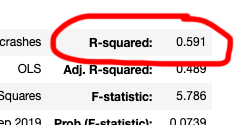• If our R squared was 1.0, 100% of the change in crashes can be explained by the number of miles
• If our R squared is 0.0, 0% of the change in crashes can be explained by the number of miles

In this case, our R squared is 0.591, which means the number of miles driven counts for about 60% of how many crashes someone gets into. The other 40% can be things like weather, luck, driving skill - other things we aren't measuring here, but which might factor in.

### Comparing regressions#

Let's say we ran another regression, taking into account miles driven as well as car age. Does this new regression do a better job explaining the variation in number of crashes?

```# What effect does the number of miles driven AND the car's age have on the number of crashes?
model = smf.ols(formula='crashes ~ np.divide(miles, 1000) + car_age', data=df)
results = model.fit()
results.summary()
```
Dep. Variable: R-squared: crashes 0.620 OLS 0.367 Least Squares 2.452 Fri, 13 Dec 2019 0.234 11:21:55 -9.6168 6 25.23 3 24.61 2 nonrobust
coef std err t P>|t| [0.025 0.975] 0.0159 1.906 0.008 0.994 -6.049 6.080 1.1111 0.517 2.148 0.121 -0.535 2.757 -0.1429 0.297 -0.480 0.664 -1.089 0.804
 Omnibus: Durbin-Watson: nan 3.454 nan 0.455 -0.384 0.796 1.89 19.1

Warnings:
 Standard Errors assume that the covariance matrix of the errors is correctly specified.

If we look at R-squared, the new one has a higher value. This means the new regression explains more of the variation in crashes.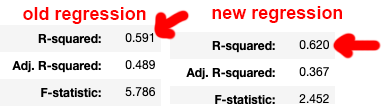An extra 2 percentage points covered! Amazing! ...except for the fact that every time you add new parameters to a regression, the R-squared is going to go up. Every single time. Every single time.

This means a regression with a thousand features is going to explain more than one with one feature, even if the extra features are only explaining random noise. As a result, no, we can't compare our regressions with R-squared.

We have a few other options to compare regressions, though! And by "a few" I mean "potentially a million."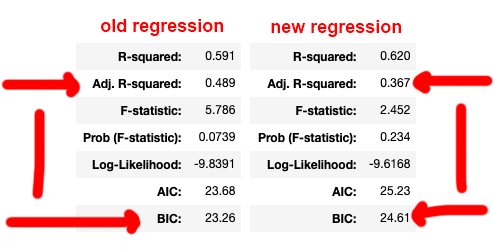You could use all of these in one way or another, but the one to pay attention to here is adjusted R-squared. It's R-squared, but adjusted for the number of features we're giving the model. For R-squared, it always goes up if you add new features. For adjusted R-squared, though, it only goes up if the extra parameters are useful.

If adjusted R-squared goes up, we (probably) have a better model.

"What about the other things in that list? AIC? BIC?" Good question: you can use all the other ones when you talk to someone who knows statistics. We'll talk about Prob (F-statistic) a little bit later, down below.

Our adjusted R-squared for the original regression was 0.489, while our adjusted R-squared for the new regression was 0.367. Since our adjusted R-squared went down with the new regression, adding `car_age` didn't actually add anything to our model!

But hey - shouldn't more data always be better data? To figure out what happened, let's look at the features themselves instead of the overall model.

## Feature quality#

Since we've figured out that adding new features to our model doesn't necessarily improve it, let's take a look at how to measure features. How can we tell a good and useful feature from a bad one?

It's easy to get excited about a big coefficient, something that claims to have a nice large effect on our output. But caution is required: features will always have a coefficient, but that doesn't mean that the coefficient is valid. I can tell you all day every day that my uncle works for Nintendo, but unless he actually works there then it doesn't matter how often I say it.

Let's examine the feature descriptions from the statsmodels output, all those rows down at the bottom. We're interested in `P>|t|`, which is the feature's p-value.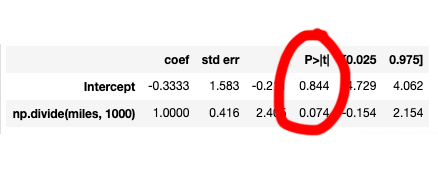The p-value is the most common way to talk about whether a feature (and its coefficient) is meaningful or not. P-values are commonly described as the chance that a result was just a lucky/unlucky accident.

The standard measure of p-value validity is 0.05, which a layman might describe as "we'd get this result accidentally only 5% of the time." It's what people usually mean when they refer to statistical significance.

Note: P-values have plenty of flaws and the "accidental results" description isn't really the most rigorous, but we're going to live with it.

If we look at the p-value for our miles, it's 0.074, which does NOT MEET THE 0.05 THRESHOLD FOR STATISTICAL SIGNIFICANCE!!! It's saying there's a 7.4% chance of this just being an accident, as opposed to under a 5% chance. Horrifying, terrifying, really.

...horrifying except for the fact that 0.05 is a completely arbitrary (yet well-accepted) measure, and sometimes people even use 0.1 (10%) or even 0.01 (1%) instead! So depending on how we're feeling when we wake up in the morning, we can totally feel free to use this. See the 'discussion topics' section for a bit more on this topic.

Our data is very small and very fake, so let's not stress out too much here.

## Change in p-values#

Let's step back to our two different regression, one that was just miles and one that was miles and the age of the car. When we compare the outputs, the p value for miles changed dramatically between the old and new regressions.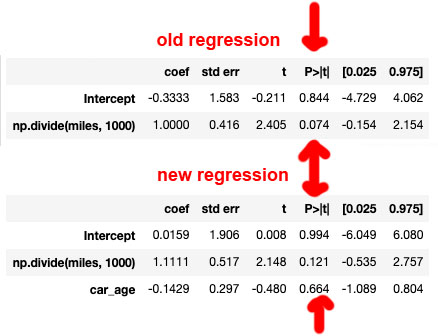The p-value for `miles` jumped from 0.074 to 0.121 when we moved to the new regression, and the car's age came in at a mind-boggling-high 0.664 (67%)! Remember: higher p-values are worse, they're a greater chance that the relationship to the output (the number of crashes) is just an accident.

Even if we're feeling gracious about miles, there's no way we can believe the car's age has anything to do with the number of crashes with its 0.664 p-value. Exposed! Car age's uncle does not work for Nintendo.

It's important to note that adding car age wasn't just useless, either: by including the car's age in our regression we negatively impacted the p-value of miles driven, almost doubling it! It might be tempting to add in a million and one features to make your regression "more informed," but we can see from this example that useless features just confuse the regression. Because our regression also had to take into account the car's age, it couldn't listen to `miles` as much as it should have.

As a result of adding `car_age`, the adjusted R-squared dropped and p-values went crazy. Bad regression!

Picking what features to use - "feature selection" - is a big and complicated part of machine learning. If you generally stick to only adding features that make sense and removing ones that have high p-values, you'll probably be in a good place when you present your findings to a stats person to review.

## Regression p values#

It isn't just our features that have p-values - the overall regression gets a p-value, too! This is where people who publish papers will get up on a cardboard box and exclaim "MY RESULTS ARE STATISTICALLY SIGNIFICANT!"

You can find the p-value for the entire regression in the top left, listed as Prob (F-statistic). Here is the p-value for our first regression: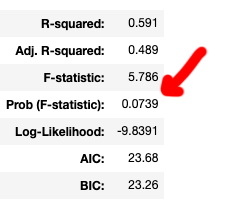In our original regression, the p-value for our overall regression is actually the same as it was for our `miles` feature, about 0.074. We can compare this p-value to the regression that included the car age: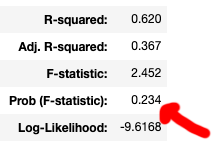Another point that shows how bad that second regression was! The p-value jumped from 0.074 to 0.234 - even if that regression had the most exciting coefficients in the world, a 0.234 p-value is way way way too high. In no universe could it be considered statistically significant, sorry!

Note that you won't use p-values to choose between models. You'll just use a p-value to determine whether the linear regression model meets your standard of statistical significance. If it doesn't, throw it out!

## Review#

In this section, we talked about evaluating both models and features.

For a linear regression model, the R-squared can be used to see how much of the output is described by the regression. Every time you add features, though, the R-squared will go up! To compare models with different numbers of features you'll need to use adjusted r-squared. Adjusted R-squared is smart enough to take into account how many features there are.

When evaluating individual features, you're typically interested in a p-value that's less than 0.05. Removing bad features can improve your model, as your regression can start paying attention to the things that matter. More data isn't necessarily better!

Models also have p values that determine whether the result can be considered "statistically significant." You won't use them to decide between models, but they're important in evaluating whether you can trust a model.

TODO

```
```